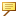Conditional Statements in RelationsRelations containing conditional statements are not sorted. A condition is an expression that is either TRUE (or YES) or FALSE (or NO). These values can be used interchangeably in the conditional statement. For example, the following statements can all be evaluated the same way:
IF ANSWER == YES
IF ANSWER == TRUE
IF Statement
You can add IF statements to relations to create conditional statements. For example,
IF d1 > d2
length = 14.5
ENDIF
IF d1 <= d2
length = 7.0
ENDIF
ELSE Statement
By adding the ELSE statements in the branches, you can create more complex conditional constructions. With these statements, the previous relation may be modified as follows:
IF d1 > d2
length = 14.5
ELSE
length = 7.0
ENDIF
There can be several features listed between the IF, ELSE, and the ENDIF statements. In addition, the IF|ELSE|ENDIF constructions can also be nested within feature sequences.
The syntax of the IF clause is as follows:
IF <condition>
Sequence of 0 or more relations or IF clauses
ELSE <optional>
Sequence of 0 or more relations or IF clauses <optional>
ENDIF
Consider the following rules:
ENDIF is spelled as one word.
ELSE is added on a separate line.
Equal in conditional statements is entered as two equal signs (==). Assignment is entered as a single equal sign (=).